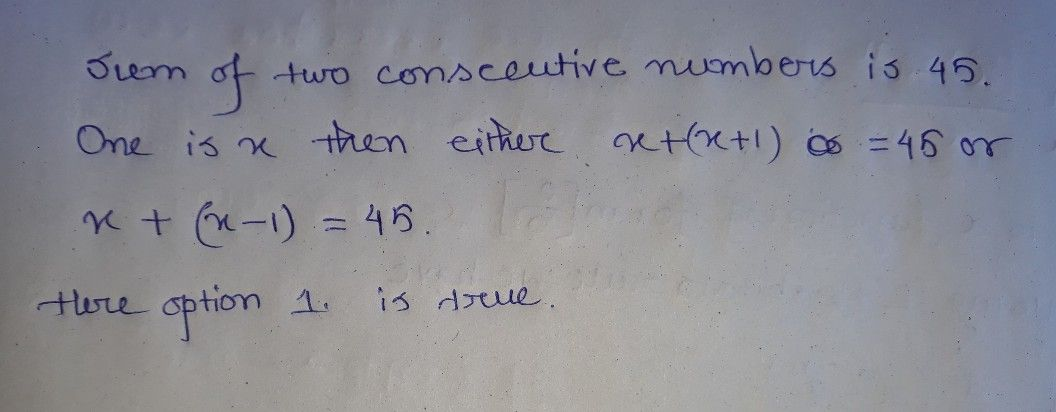Symbol
ProblemIf the sum of two consecutive numbers is $45$ and one number is $X$ .This statement in the form of equation $1s:$ $\left(1$ Point) $\right)$ $○5x+1$ $1eft\left(x+1$ $r1gnt\right)=45s$ $○sx+1ef\left(x+2$ $r1gnt\right)=145s$ $sx+1x=45s$